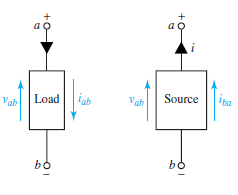Electric Potential and Voltage: When electrical forces act on a particle, it will possess potential energy. In order to describe the potential energy that a particle will have at a point x, the electric potential at point x is defined as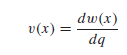where w(x) is the potential energy that a particle with charge q has when it is located at the position x. The zero point of potential energy can be chosen arbitrarily since only differences in energy have practical meaning. The point where electric potential is zero is known as the referencepoint or ground point, with respect to which potentials at other points are then described. The potential difference is known as the voltage expressed in volts (V) or joules per coulomb (J/C).If the potential at B is higher than that at A,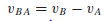which is positive. Obviously voltages can be either positive or negative numbers, and it follows that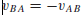The voltage at point A, designated as vA, is then the potential at point A with respect to the ground.

Energy and Power: If a charge dq gives up energy dw when going from point a to point b, then the voltage across those points is defined as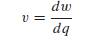If dw/dq is positive, point a is at the higher potential. The voltage between two points is the work per unit positive charge required to move that charge between the two points. If dw and dq have the same sign, then energy is delivered by a positive charge going from a to b (or a negative charge going the other way). Conversely, charged particles gain energy inside a source where dw and dq have opposite polarities.

The load and source conventions are shown in Figure, in which point a is at a higher potential than point b. The load receives or absorbs energy because a positive charge goes in the direction of the current arrow from higher to lower potential. The source has a capacity to supply energy. The voltage source is sometimes known as an electromotive force, or emf, to convey the notation that it is a force that drives the current through the
circuit. The instantaneous power p is defined as the rate of doing work or the rate of change of energy dw/dt,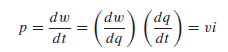The electric power consumed or produced by a circuit element is given by its voltage–current product, expressed in volt-amperes (VA) or watts (W). The energy over a time interval is found by integrating power,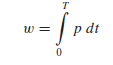which is expressed in watt-seconds or joules (J), or commonly in electric utility bills in kilowatthours (kWh). Note that 1 kWh equals 3.6 × 106 J.